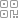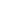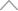5 9 7 0 4 4 3

# 队列，帮我搞一下这个滑动窗口最大值。

• 滑动 ：窗口可以按照一定的方向移动。

• 窗口 ：窗口大小可以固定，也可以不固定，此时可以向外或者向内，扩容或者缩小窗口直至满足条件。LeetC ode 239：滑动窗口最大值1 <= nums.length <= 10^5

-10^4 <= nums[i] <= 10^4

1 <= k <= nums.length# 如果数组为空或 k = 0，直接返回空

if not nums or not k:

return []

# 如果数组只有1个元素，直接返回该元素

if len(nums) == 1:

return [nums]

# 初始化队列和结果，队列存储数组的下标

queue = []

res = []# 对于新进入的元素，如果队列前面的数比它小，那么前面的都出队列

while queue and nums[queue[-1]] < nums[i]:

queue.pop()

# 新元素入队列

queue.append(i)# 当前的大值加入到结果数组中

if i >= k-1:

res.append(nums[queue])# 如果当前队列最左侧存储的下标等于 i-k 的值，代表目前队列已满。

# 但是新元素需要进来，所以列表最左侧的下标出队列

if queue and queue == i - k:

queue.pop(0)Python 代码实现

class Solution:

def maxSlidingWindow(self, nums: List[int], k: int) -> List[int]:

# 如果数组为空或 k = 0，直接返回空

if not nums or not k:

return []

# 如果数组只有1个元素，直接返回该元素

if len(nums) == 1:

return [nums]

# 初始化队列和结果，队列存储数组的下标

queue = []

res = []

for i in range(len(nums)):

# 如果当前队列最左侧存储的下标等于 i-k 的值，代表目前队列已满。

# 但是新元素需要进来，所以列表最左侧的下标出队列

if queue and queue == i - k:

queue.pop(0)

# 对于新进入的元素，如果队列前面的数比它小，那么前面的都出队列

while queue and nums[queue[-1]] < nums[i]:

queue.pop()

# 新元素入队列

queue.append(i)

# 当前的大值加入到结果数组中

if i >= k-1:

res.append(nums[queue])

return res

Java 代码实现

class Solution {

public int[] maxSlidingWindow(int[] nums, int k) {

if(nums == null || nums.length < 2) return nums;

// 双端队列 保存当前窗口最大值的数组位置 保证队列中数组位置的数值按从大到小排序

// 结果数组

int[] result = new int[nums.length-k+1];

for(int i = 0;i < nums.length;i++){

// 保证从大到小 如果前面数小则需要依次弹出，直至满足要求

while(!queue.isEmpty() && nums[queue.peekLast()] <= nums[i]){

queue.pollLast();

}

// 添加当前值对应的数组下标

// 判断当前队列中队首的值是否有效

if(queue.peek() <= i-k){

queue.poll();

}

// 当窗口长度为k时 保存当前窗口中最大值

if(i+1 >= k){

result[i+1-k] = nums[queue.peek()];

}

}

return result;

}

}

AC 不是目的，目的是好好去琢磨琢磨这种解题方法，然后能运用到之后的题目中。

## 新知精选

IT研发知识▪优秀创作者

### 优选课程 新知学院

•扫码下载商业新知APP~

•回到顶部